All About Science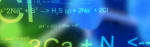# General Relativity

QUESTION: What is General Relativity?

ANSWER:

General relativity is also referred to as "The General Theory of Relativity." It was initially presented in a paper by Albert Einstein in 1915. Its primary thrust was to add the effects of gravity to "The Special Theory of Relativity," making special relativity a special case of general relativity. In the same way, ten years earlier, Einstein proposed The Theory of Special Relativity with the primary thrust of eliminating the concept of a fixed reference frame in favor of relative inertial frames in conjunction with the newly learned fact that the speed of light was a constant when measured in any inertial reference frame. This theory, in a similar way, makes the Newtonian Euclidian geometry of space a special case of special relativity. So rather than these new theories refuting the old theories, they actually verified that the previous theories were special cases of a more complicated theory that explains more of reality.

Many of us who have studied physics remember an equation that states that force equals mass times acceleration (f = ma) for a mass being accelerated by a constant force. We also remember how strange it was that an almost identical equation, force equals mass times the gravitational acceleration constant (f = mg) was used to determine the weight of a non-accelerating object in a gravitational field. This similarity (or relationship) between "a" and "g" forms the conceptual basis for general relativity.

Consider yourself standing on a scale in an enclosed elevator at rest. Also consider yourself standing on a similar scale in an enclosed spaceship accelerating at one "g." Both the equation and our intuitive knowledge tell us that we cannot distinguish between the one situation and the other. We would be standing balanced on the scale with the scale reading our weight in both cases. Also consider the opposite situation where the elevator was in free fall and the spaceship was way out in space away from any star with the rockets off. In both cases, again, we cannot distinguish between being in the elevator or the spaceship. In both cases, the scale would read zero and we would be floating with no forces on our body.

Now, make two small windows in the opposite sides of both the elevator and spaceship so a line between the windows is perpendicular to the normal direction of movement. Consider the last case where the elevator is in free fall and the spaceship is floating in space. It is obvious that the light beam would go straight through the windows of the spaceship. It is argued that since we cannot distinguish between the spaceship at rest and the elevator in freefall, light would also go straight through the two windows of the elevator. Now consider the opposite scenario where the elevator is at rest on earth and the spaceship is being accelerated at the rate of one "g." For the spaceship, the light beam would curve as it goes between the two windows because as the beam propagates across the spaceship, the spaceship is being accelerated. It is argued that since we cannot distinguish between the accelerated spaceship and the elevator at rest, light bends between the windows of the elevator also. This means, if you are at rest in a gravitational field, light is deflected. So a gravitational field deflects or bends light.

Based on this conceptual foundation, additional general relativity concepts and effects have been developed. One concept is the effect of gravitational fields to cause the space-time continuum to be curved or warped by large masses. Another effect is that gravity, in addition to bending light, also can cause it to slow down. This slowing down of light results in a time dilation effect where time actually slows up. The slowing down effect of earth's gravity on time at its surface is very little. Compared with space, the earth's gravitational field slows time by only one second per billion seconds. Experiments have verified the validity of general relativity. Some of these experiments include the precession of the perihelion of Mercury, the deflection of light, and the gravitational red shift of light.

Although the earth with its low gravitational field is at one end of the spectrum, black holes predicted by general relativity and observed in space have extremely high gravity and are at the other end of the spectrum. Black holes, in addition to bending light, slowing down light, and slowing down time, can stop light from escaping and make time stand still. For us, watching a space probe speeding toward the event horizon of a black hole, the space probe would appear to slow down and virtually stop. The fact that science can show that time can virtually stop helps our understanding of how a transcendent God, beyond time and space, can exist.

Learn More about the Theory of Relativity!

####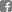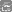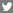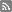Copyright © 2002-2019 AllAboutScience.org, All Rights Reserved